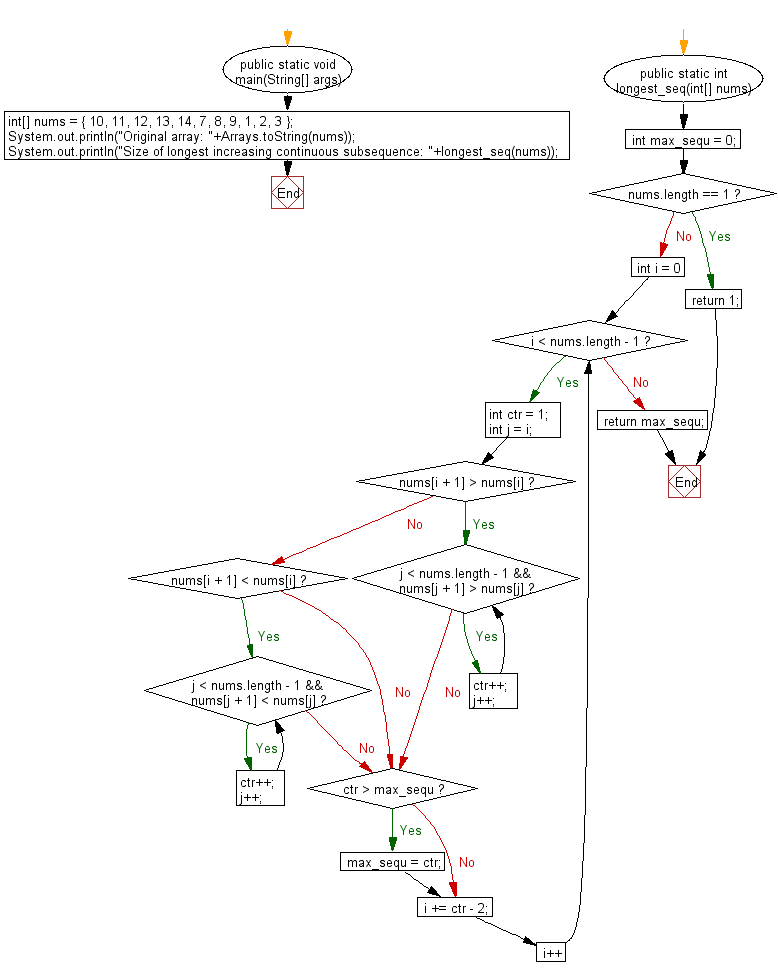﻿ Java programming exercises: Find the longest increasing continuous subsequence in a given array of integers - w3resource# Java Exercises: Find the longest increasing continuous subsequence in a given array of integers

## Java Basic: Exercise-178 with Solution

Write a Java program to find the longest increasing continuous subsequence in a given array of integers.

Sample Solution:

Java Code:

``````import java.util.*;
public class Solution {
public static void main(String[] args) {
int[] nums = { 10, 11, 12, 13, 14, 7, 8, 9, 1, 2, 3 };
System.out.println("Original array: " + Arrays.toString(nums));
System.out.println("Size of longest increasing continuous subsequence: " + longest_seq(nums));
}

public static int longest_seq(int[] nums) {
int max_sequ = 0;
if (nums.length == 1) return 1;
for (int i = 0; i < nums.length - 1; i++) {
int ctr = 1;
int j = i;
if (nums[i + 1] > nums[i]) {
while (j < nums.length - 1 && nums[j + 1] > nums[j]) {
ctr++;
j++;
}
} else if (nums[i + 1] < nums[i]) {
while (j < nums.length - 1 && nums[j + 1] < nums[j]) {
ctr++;
j++;
}
}
if (ctr > max_sequ) {
max_sequ = ctr;
}
i += ctr - 2;
}
return max_sequ;
}
}
```
```

Sample Output:

```Original array: [10, 11, 12, 13, 14, 7, 8, 9, 1, 2, 3]
Size of longest increasing continuous subsequence: 5
```

Pictorial Presentation:Flowchart:Java Code Editor:

Company:

What is the difficulty level of this exercise?

﻿

## Java: Tips of the Day

Array vs ArrayLists:

The main difference between these two is that an Array is of fixed size so once you have created an Array you cannot change it but the ArrayList is not of fixed size. You can create instances of ArrayLists without specifying its size. So if you create such instances of an ArrayList without specifying its size Java will create an instance of an ArrayList of default size.

Once an ArrayList is full it re-sizes itself. In fact, an ArrayList is internally supported by an array. So when an ArrayList is resized it will slow down its performance a bit as the contents of the old Array must be copied to a new Array.

At the same time, it's compulsory to specify the size of an Array directly or indirectly while creating it. And also Arrays can store both primitives and objects while ArrayLists only can store objects.

Ref: https://bit.ly/3o8L2KH